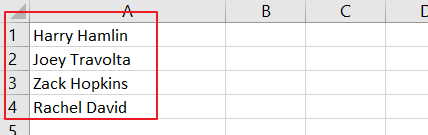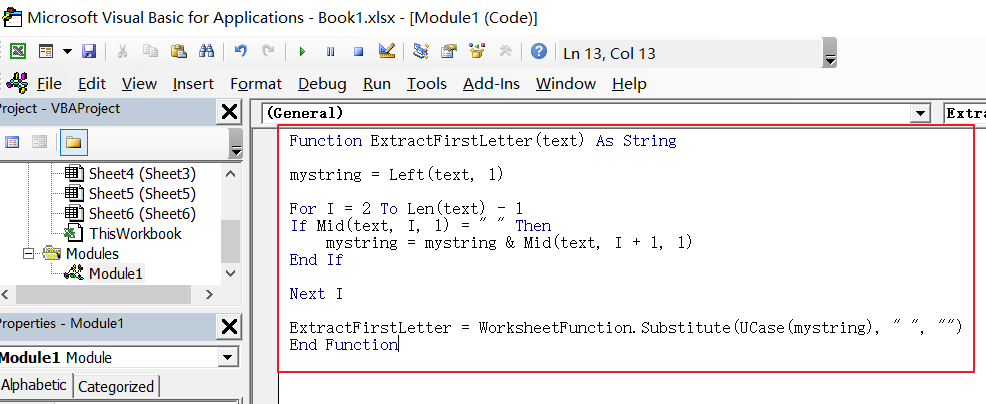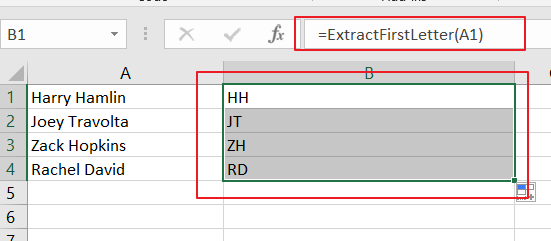# How to Extract First Letter from Each Word in a Cell in Excel

This post will guide you how to extract first letter from each word in a given cell in Excel. How do I extract the first letter of each word in a range of names in your current worksheet in Microsoft Excel 2013/2016. Assume that you have a range of First, Second and third names and you would like to extract the initials to a separate cell.

For example, one name called “Nigolas Kate”, and you want to extract the first letters so that the result would be “NK”.Since there is not built-in function to extract the first letter of each word in Excel, and you can define a user defined function by Excel VBA code to achieve the result. Just do the following steps:

#1 open your excel workbook and then click on “Visual Basic” command under DEVELOPER Tab, or just press “ALT+F11” shortcut.#2 then the “Visual Basic Editor” window will appear.

#3 click “Insert” ->”Module” to create a new module.#4 paste the below VBA code into the code window. Then clicking “Save” button.

```Function ExtractFirstLetter(text) As String

mystring = Left(text, 1)

For i = 2 To Len(text) - 1
If Mid(text, i, 1) = " " Then
mystring = mystring & Mid(text, i + 1, 1)
End If

Next i

ExtractFirstLetter = WorksheetFunction.Substitute(UCase(mystring), " ", "")
End Function```#5 back to the current worksheet, then type the following formula in a blank cell, and then press Enter key.

=ExtractFirstLetter(A1)Sidebar